# Tag: lattices

Sunday, January 28th 1940, Hamburg

Ernst Witt wants to get two papers out of his system because he knows he’ll have to enter the Wehrmacht in February.

The first one, “Spiegelungsgruppen und Aufzählung halbeinfacher Liescher Ringe”, contains his own treatment of the root systems of semisimple Lie algebras and their reflexion groups, following up on previous work by Killing, Cartan, Weyl, van der Waerden and Coxeter.(Photo: Natascha Artin, Nikolausberg 1933): From left to right: Ernst Witt; Paul Bernays; Helene Weyl; Hermann Weyl; Joachim Weyl, Emil Artin; Emmy Noether; Ernst Knauf; unknown woman; Chiuntze Tsen; Erna Bannow (later became wife of Ernst Witt)

Important for our story is that this paper contains the result stating that integral lattices generated by norm 2 elements are direct sums of root systems of the simply laced Dynkin diagrans $A_n, D_n$ and $E_6,E_7$ or $E_8$ (Witt uses a slightly different notation).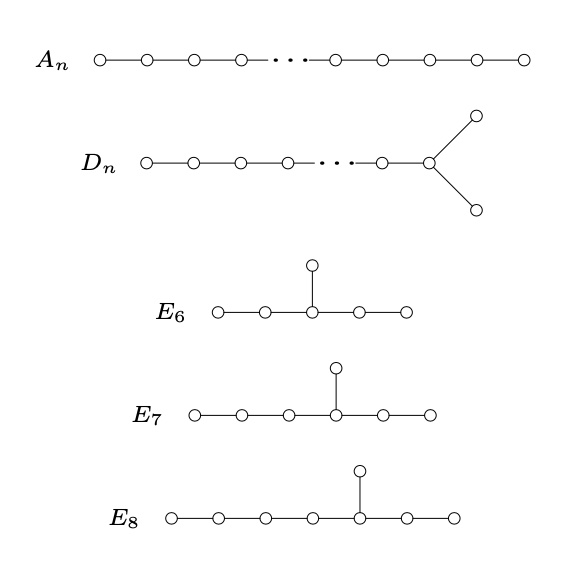In each case, Witt knows of course the number of roots and the determinant of the Gram matrix
$\begin{array}{c|cc} & \# \text{roots} & \text{determinant} \\ \hline A_n & n^2+n & n+1 \\ D_n & 2n^2-2n & 4 \\ E_6 & 72 & 3 \\ E_7 & 126 & 2 \\ E_8 & 240 & 1 \end{array}$
The second paper “Eine Identität zwischen Modulformen zweiten Grades” proves that there are just two positive definite even unimodular lattices (those in which every squared length is even, and which have one point per unit volume, that is, have determinant one) in dimension sixteen, $E_8 \oplus E_8$ and $D_{16}^+$. Previously, Louis Mordell showed that the only unimodular even lattice in dimension $8$ is $E_8$.

The connection with modular forms is via their theta series, listing the number of lattice points of each squared length
$\theta_L(q) = \sum_{m=0}^{\infty} \#\{ \lambda \in L : (\lambda,\lambda)=m \} q^{m}$
which is a modular form of weight $n/2$ ($n$ being the dimension which must be divisible by $8$) in case $L$ is a positive definitive even unimodular lattice.

The algebra of all modular forms is generated by the Eisenstein series $E_2$ and $E_3$ of weights $4$ and $6$, so in dimension $8$ we have just one possible theta series
$\theta_L(q) = E_2^2 = 1+480 q^2+ 61920 q^4+ 1050240 q^6+ \dots$

It is interesting to read Witt’s proof of his main result (Satz 3) in which he explains how he constructed the possible even unimodular lattices in dimension $16$.

He knows that the sublattice of $L$ generated by the $480$ norm two elements must be a direct sum of root lattices. His knowledge of the number of roots in each case tells him there are only two possibilities
$E_8 \oplus E_8 \qquad \text{and} \qquad D_{16}$
The determinant of the Gram matrix of $E_8 \oplus E_8$ is one, so this one is already unimodular. The remaining possibility
$D_{16} = \{ (x_1,\dots,x_{16}) \in \mathbb{Z}^{16}~|~x_1+ \dots + x_{16} \in 2 \mathbb{Z} \}$
has determinant $4$ so he needs to add further lattice points (necessarily contained in the dual lattice $D_{16}^*$) to get it unimodular. He knows the coset representatives of $D_{16}^*/D_{16}$:
$\begin{cases} =(0, \dots,0) &~\text{of norm 0} \\ =(\tfrac{1}{2},\dots,\tfrac{1}{2}) &~\text{of norm 4} \\ =(0,\dots,0,1) &~\text{of norm 1} \\ =(\tfrac{1}{2},\dots,\tfrac{1}{2},-\tfrac{1}{2}) &~\text{of norm 4} \end{cases}$
and he verifies that the determinant of $D_{16}^+=D_{16}+(+D_{16})$ is indeed one (btw. adding coset $$ gives an isomorphic lattice). Witt calls this procedure to arrive at the correct lattices forced (‘zwangslaufig’).

So, how do you think Witt would go about finding even unimodular lattices in dimension $24$?

To me it is clear that he would start with a direct sum of root lattices whose dimensions add up to $24$, compute the determinant of the Gram matrix and, if necessary, add coset classes to arrive at a unimodular lattice.

Today we would call this procedure ‘adding glue’, after Martin Kneser, who formalised this procedure in 1967.

On January 28th 1940, Witt writes that he found more than $10$ different classes of even unimodular lattices in dimension $24$ (without giving any details) and mentioned that the determination of the total number of such lattices will not be entirely trivial (‘scheint nicht ganz leicht zu sein’).

The complete classification of all $24$ even unimodular lattices in dimension $24$ was achieved by Hans Volker Niemeier in his 1968 Ph.D. thesis “Definite quadratische Formen der Dimension 24 und Diskriminante 1”, under the direction of Martin Kneser. Naturally, these lattices are now known as the Niemeier lattices.

Which of the Niemeier lattices were known to Witt in 1940?

There are three obvious certainties: $E_8 \oplus E_8 \oplus E_8$, $E_8 \oplus D_{16}^+$ (both already unimodular, the second by Witt’s work) and $D_{24}^+$ with a construction analogous to the one of $D_{16}^+$.

To make an educated guess about the remaining Witt-Niemeier lattices we can do two things:

1. use our knowledge of Niemeier lattices to figure out which of these Witt was most likely to stumble upon, and
2. imagine how he would adapt his modular form approach in dimension $16$ to dimension $24$.

Here’s Kneser’s neighbourhood graph of the Niemeier lattices. Its vertices are the $24$ Niemeiers and there’s an edge between $L$ and $M$ whenever the intersection $L \cap M$ is of index $2$ in both $L$ and $M$. In this case, $L$ and $M$ are called neighbours.Although the theory of neighbours was not known to Witt, the graph may give an indication of how likely it is to dig up a new Niemeier lattice by poking into an already discovered one.
The three certainties are the three lattices at the bottom of the neighborhood graph, making it more likely for the lattices in the lower region to be among Witt’s list.

For the other approach, the space of modular forms of weight $12$ is two dimensional, with a basis formed by the series
$\begin{cases} E_6(q) = 1 + \tfrac{65520}{691}(q+2049 q^2 + 177148 q^3+4196353q^4+\dots \\ \Delta(q) = q-24 q^2+252q^3-1472q^4+ \dots \end{cases}$

If you are at all with me, Witt would start with a lattice $R$ which is a direct sum of root lattices, so he would know the number of its roots (the norm $2$ vectors in $R$), let’s call this number $r$. Now, he wants to construct an even unimodular lattice $L$ containing $R$, so the theta series of both $L$ and $R$ must start off with $1 + r q^2 + \dots$. But, then he knows
$\theta_L(q) = E_6(q) + (r-\frac{65520}{691})\Delta(q)$
and comparing coefficients of $\theta_L(q)$ with those of $\theta_R(q)$ will give him an idea what extra vectors he has to throw in.

If we’re generous to Witt (and frankly, why shouldn’t we), we may believe that he used his vast knowledge of Steiner systems (a few years earlier he wrote the definite paper on the Mathieu groups, and a paper on Steiner systems) to construct in this way the lattices $(A_1^{24})^+$ and $(A_2^{12})^+$.

The ‘glue’ for $(A_1^{24})^+$ is coming from the extended binary Golay code, which itself uses the Steiner system $S(5,8,24)$. $(A_2^{12})^+$ is constructed using the extended ternary Golay code, based on the Steiner system $S(5,6,12)$.

The one thing that would never have crossed his mind that sunday in 1940 was to explore the possibility of an even unimodular 24-dimensional lattice $\Lambda$ without any roots!

One with $r=0$, and thus with a theta series starting off as
$\theta_{\Lambda}(q) = 1 + 196560 q^4 + 16773120 q^6 + \dots$
No, he did not find the Leech lattice that day.

If he would have stumbled upon it, it would have simply blown his mind.

It would have been so much against all his experiences and intuitions that he would have dropped everything on the spot to write a paper about it, or at least, he would have mentioned in his ‘more than $10$ lattices’-claim that, surprisingly, one of them was an even unimodular lattice without any roots.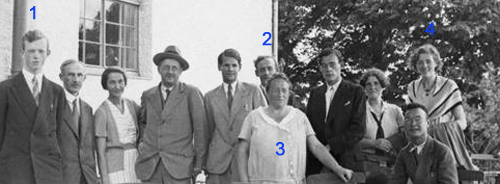For the better part of the 30ties, Ernst Witt (1) did hang out with the rest of the ‘Noetherknaben’, the group of young mathematicians around Emmy Noether (3) in Gottingen.

In 1934 Witt became Helmut Hasse‘s assistent in Gottingen, where he qualified as a university lecturer in 1936. By 1938 he has made enough of a name for himself to be offered a lecturer position in Hamburg and soon became an associate professor, the down-graded position held by Emil Artin (2) until he was forced to emigrate in 1937.

A former fellow student of him in Gottingen, Erna Bannow (4), had gone earlier to Hamburg to work with Artin. She continued her studies with Witt and finished her Ph.D. in 1939. In 1940 Erna Bannow and Witt married.

So, life was smiling on Ernst Witt that sunday january 28th 1940, both professionally and personally. There was just one cloud on the horizon, and a rather menacing one. He was called up by the Wehrmacht and knew he had to enter service in february. For all he knew, he was spending the last week-end with his future wife… (later in february 1940, Blaschke helped him to defer his military service by one year).

Still, he desperately wanted to finish his paper before entering the army, so he spend most of that week-end going through the final version and submitted it on monday, as the published paper shows.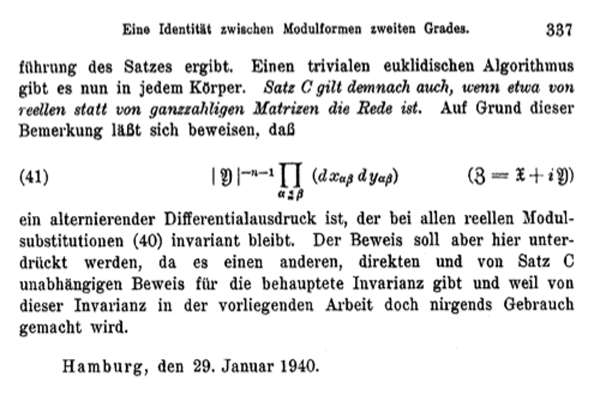In the 70ties, Witt suddenly claimed he did discover the Leech lattice ${\Lambda}$ that sunday. Last time we have seen that the only written evidence for Witt’s claim is one sentence in his 1941-paper Eine Identität zwischen Modulformen zweiten Grades. “Bei dem Versuch, eine Form aus einer solchen Klassen wirklich anzugeben, fand ich mehr als 10 verschiedene Klassen in ${\Gamma_{24}}$.”

But then, why didn’t Witt include more details of this sensational lattice in his paper?

Ina Kersten recalls on page 328 of Witt’s collected papers : “In his colloquium talk “Gitter und Mathieu-Gruppen” in Hamburg on January 27, 1970, Witt said that in 1938, he had found nine lattices in ${\Gamma_{24}}$ and that later on January 28, 1940, while studying the Steiner system ${S(5,8,24)}$, he had found two additional lattices ${M}$ and ${\Lambda}$ in ${\Gamma_{24}}$. He continued saying that he had then given up the tedious investigation of ${\Gamma_{24}}$ because of the surprisingly low contribution

$\displaystyle | Aut(\Lambda) |^{-1} < 10^{-18}$

to the Minkowski density and that he had consented himself with a short note on page 324 in his 1941 paper.”

In the last sentence he refers to the fact that the sum of the inverse orders of the automorphism groups of all even unimodular lattices of a given dimension is a fixed rational number, the Minkowski-Siegel mass constant. In dimension 24 this constant is

$\displaystyle \sum_{L} \frac{1}{| Aut(L) |} = \frac {1027637932586061520960267}{129477933340026851560636148613120000000} \approx 7.937 \times 10^{-15}$

That is, Witt was disappointed by the low contribution of the Leech lattice to the total constant and concluded that there might be thousands of new even 24-dimensional unimodular lattices out there, and dropped the problem.

If true, the story gets even better : not only claims Witt to have found the lattices ${A_1^{24}=M}$ and ${\Lambda}$, but also enough information on the Leech lattice in order to compute the order of its automorphism group ${Aut(\Lambda)}$, aka the Conway group ${Co_0 = .0}$ the dotto-group!

Is this possible? Well fortunately, the difficulties one encounters when trying to compute the order of the automorphism group of the Leech lattice from scratch, is one of the better documented mathematical stories around.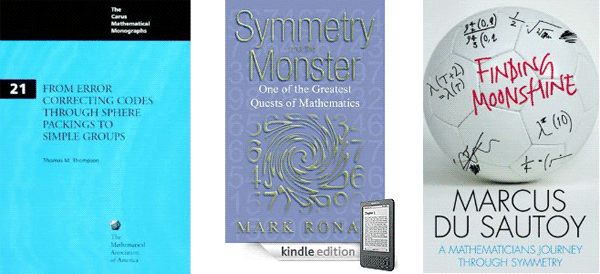The books From Error-Correcting Codes through Sphere Packings to Simple Groups by Thomas Thompson, Symmetry and the monster by Mark Ronan, and Finding moonshine by Marcus du Sautoy tell the story in minute detail.

It took John Conway 12 hours on a 1968 saturday in Cambridge to compute the order of the dotto group, using the knowledge of Leech and McKay on the properties of the Leech lattice and with considerable help offered by John Thompson via telephone.

But then, John Conway is one of the fastest mathematicians the world has known. The prologue of his book On numbers and games begins with : “Just over a quarter of a century ago, for seven consecutive days I sat down and typed from 8:30 am until midnight, with just an hour for lunch, and ever since have described this book as “having been written in a week”.”

Conway may have written a book in one week, Ernst Witt did complete his entire Ph.D. in just one week! In a letter of August 1933, his sister told her parents : “He did not have a thesis topic until July 1, and the thesis was to be submitted by July 7. He did not want to have a topic assigned to him, and when he finally had the idea, he started working day and night, and eventually managed to finish in time.”

So, if someone might have beaten John Conway in fast-computing the dottos order, it may very well have been Witt. Sadly enough, there is a lot of circumstantial evidence to make Witt’s claim highly unlikely.

For starters, psychology. Would you spend your last week-end together with your wife to be before going to war performing an horrendous calculation?

Secondly, mathematical breakthroughs often arise from newly found insight. At that time, Witt was also working on his paper on root lattices “Spiegelungsgrupen and Aufzähling halbeinfacher Liescher Ringe” which he eventually submitted in january 1941. Contained in that paper is what we know as Witt’s lemma which tells us that for any integral lattice the sublattice generated by vectors of norms 1 and 2 is a direct sum of root lattices.

This leads to the trick of trying to construct unimodular lattices by starting with a direct sum of root lattices and ‘adding glue’. Although this gluing-method was introduced by Kneser as late as 1967, Witt must have been aware of it as his 16-dimensional lattice ${D_{16}^+}$ is constructed this way.

If Witt wanted to construct new 24-dimensional even unimodular lattices in 1940, it would be natural for him to start off with direct sums of root lattices and trying to add vectors to them until he got what he was after. Now, all of the Niemeier-lattices are constructed this way, except for the Leech lattice!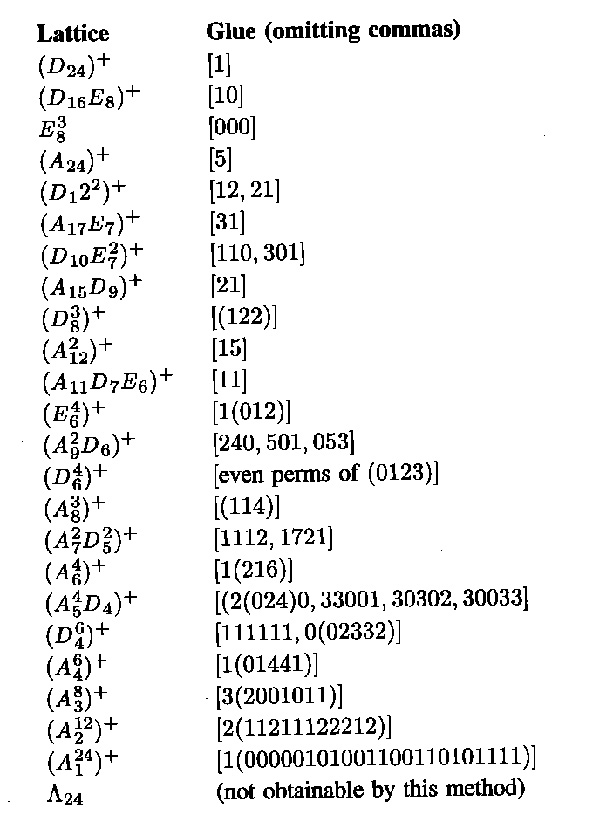I’m far from an expert on the Niemeier lattices but I would say that Witt definitely knew of the existence of ${D_{24}^+}$, ${E_8^3}$ and ${A_{24}^+}$ and that it is quite likely he also constructed ${(D_{16}E_8)^+, (D_{12}^2)^+, (A_{12}^2)^+, (D_8^3)^+}$ and possibly ${(A_{17}E_7)^+}$ and ${(A_{15}D_9)^+}$. I’d rate it far more likely Witt constructed another two such lattices on sunday january 28th 1940, rather than discovering the Leech lattice.

Finally, wouldn’t it be natural for him to include a remark, in his 1941 paper on root lattices, that not every even unimodular lattices can be obtained from sums of root lattices by adding glue, the Leech lattice being the minimal counter-example?

If it is true he was playing around with the Steiner systems that sunday, it would still be a pretty good story he discovered the lattices ${(A_2^{12})^+}$ and ${(A_1^{24})^+}$, for this would mean he discovered the Golay codes in the process!

Which brings us to our next question : who discovered the Golay code?